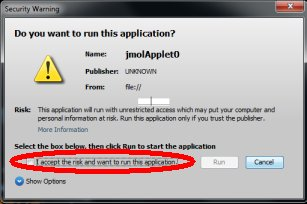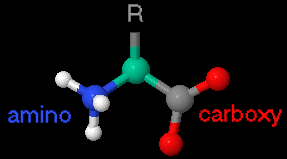If prompted, allow your browser to view blocked content. The OMM now uses the Signed Jmol Applet. No files from this site can damage your computer. Just check the appropriate box and then click run.## Notes: 1) loading of all molecules may take some time; 2) molecules are not all shown at the same scale

### Backbones are oriented amino (left) to carboxy (right)### Name colors indicate:hydrophobic,polar (non-charged),polar (charged-basic),polar (charged-acidic)

 alanine (ala = A) rotate 360o valine (val = V) rotate 360o leucine (leu = L) rotate 360o isoleucine (ile = I) rotate 360o methionine (met = M) rotate 360o proline (pro = P) rotate 360o phenylalanine (phe = F) rotate 360o tryptophan (trp = W) rotate 360o glycine (gly = G) rotate 360o serine (ser = S) rotate 360o threonine (thr = T) rotate 360o glutamine (gln = Q) rotate 360o asparagine (asn = N) rotate 360o cysteine (cys = C) rotate 360o tyrosine (tyr = Y) rotate 360o glutamic acid (glu = E) rotate 360o aspartic acid (asp = D) rotate 360o arginine (arg = R) rotate 360o lysine (lys = K) rotate 360o histidine (his = H) rotate 360o